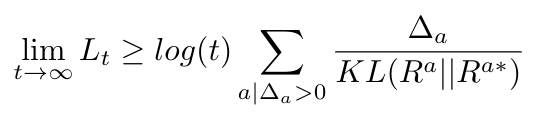# The Silver Challenge - Lecture 9

Today’s lecture discussed more intelligent methods of trading off exploration and exploitation than epsilon-greedy, which is the only method we have seen so far . Silver reviewed three approaches to making this trade-off, and he explained that for all of them, there is a lower bound on how well they can perform . I’m going to briefly explain that lower bound and then introduce the idea behind one of these three approaches which I thought was particularly interesting.

## Lai and Robbins Theorem

This theorem states that the asymptotic total regret (i.e. total missed opportunity for an exploration-exploitation trade-off algorithm) is at least logarithmic in the number of steps :Equation 1

Here the log(t) term represents the logarithmic limit over the number of states . The numerator in the fraction represents the gap between different actions that we can take . A larger gap means that two actions have very different means, and so if we pick the worse-performing action, we incur a large regret . The denominator, the KL-divergence, is a measure of the similarity of the distributions . If the KL-divergence is small, it means that the distribution of the rewards over the two actions looks very similar, and this can increase the amount of regret we accrue . Difficult problems have small values of the KL-divergence because it means that we have a very difficult problem where the two actions appear to have very similar distributions . Difficult problems also have large gaps which indicate that the real means between the two actions are very different, and so choosing the wrong action can incur a large penalty .

## Optimism in the Face of Uncertainty

One of the three approaches to balancing the exploration/exploitation dilemma was called choosing optimism in the face of uncertainty . I thought it was an interesting idea that we had not yet seen, because instead of assuming that unknown states have zero reward until proven otherwise, with optimism in the face of uncertainty, we actually assume that we have maximum reward until proven otherwise . The result of this assumption is that we are motivated to visit the states we know the least about (because they were initialized with the highest reward) and we progressively decrement those states rewards until they reflect their true rewards . This method drives us to frequently choose the states that are the most unknown if there is a possibility that they could yield a higher reward than any of our known states .

 Silver, D. “RL Course by David Silver - Lecture 9: Exploration and Exploitation.” YouTube. 13 May 2015. https://www.youtube.com/watch?v=sGuiWX07sKw&list=PLqYmG7hTraZDM-OYHWgPebj2MfCFzFObQ&index=9 Visited 14 Aug 2020.